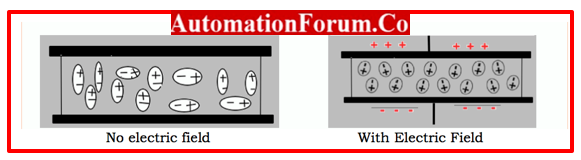# Permittivity vs Permeability## Permittivity and Permeability

In electromagnetism, permeability and permittivity are two separate metrics. A material’s capacity to store energy is permittivity. A material’s permeability is a measurement of how well it can support the creation of an internal magnetic field.

## Permittivity

### Define Permittivity?

The material or medium has a property called permittivity that measures the resistance for an electric field. The permittivity can be described as ratio of Electric Displacement Field to Electric field applied.

Permittivity Expressed as ε= D/ E  (Farad/meter)

• D = Electric Displacement field
• E = Electric Field Applied
• When there is a better polarization then the resistivity is also better.
• Higher permittivity the resistance is high and Lower permittivity the resistance is low

### Types of Permittivity & Relation between them

#### Absolute Permittivity (ε)

• Absolute permittivity is the property of the medium that determines how much flux density is produced at a certain point inside the field and specifies a particular electric field intensity at that location in the field.
• It is possible to represent the absolute permittivity of any medium by multiplying the permittivity of the vacuum with the relative permittivity of the medium. This is known as the product of these two numbers.

#### Vacuum Permittivity (ε0)

The permittivity of free space is known as vacuum permittivity. It is referred by the symbol ε0. Its value is 8.85 x 10-12 Fm-1

#### Relative Permittivity (εr)

Relative permittivity is referred as dielectric constant, is the ratio of the absolute permittivity of a material to the vacuum permittivity.

The relationship can be expressed as

εr = ε/ ε0 .

### What are the various parameters the permittivity depends on?

The permittivity of a material depends on

• Type of the medium or the material
• Strength of the electric field
• Frequency of the applied electric field
• Humidity
• Temperature

### Dielectric properties of different materials

• The minimal value of permittivity is represented by the permittivity of free space.
• All other materials and substances have a relative permittivity greater than 1.
• These compounds are referred to as dielectrics or dielectric materials.
• A substance with a dielectric constant larger than 1 and low conductivity is referred to as a dielectric.
• Glass, paper, mica, different ceramics, polyethylene, and several metal oxides are examples of common dielectrics.

## Permeability

### What is meant by Permeability?

• Permeability is the capacity of a substance or medium to become magnetized when exposed to an external magnetic field.
• Also known as magnetic permeability.
• When contrasted to the magnetizing field in which the given material is put, it is the relative increase or reduction in the magnetic field that results as a result inside the material.

Magnetic permeability is denoted as μ

μ = B/H [Henry/meter]

where

• μ = Absolute permeability
• B = Magnetic Flux Density
• H = Magnetic Field Strength
• When permeability is high, conductivity is also high.
• If permeability is low, conductivity will be too.

### Types of Permeability & Relation between them

#### Relative Permeability

The ratio between the flux density produced in a material and the flux density produced in a vacuum by the same magnetizing force is the measure of a material’s relative permeability.

Represented as μr = B/Bo

• B = Flux Density in the material
• B0 = Flux Density in a vacuum

#### Absolute Permeability

Permeability in free space is represented as μ0is called as absolute permeability. Its value is 4∏× 10-7 H/m. This permeability value is used as a benchmark and is considered to be a constant.

Relation between Permeability, Absolute permeability & Relative Permeability

μr=μ/μo

### What are the various parameters the permeability depends on?

The factors that varies the permeability are

• Strength of the magnetic field
• Position of material in the magnetic field
• Humidity
• Temperature
• Frequency of the applied field

### Classification of Materials as per Permeability

• Diamagnetic: If μr is less than 1, this material has opposition to external magnetic field. Example: Copper, Zinc, Marble, Glass, Gold
• Paramagnetic: Ifμr is nearly about 1 but not 1 exactly, the material is weakly attracted by external magnetic field.Example: Titanium, Aluminum, Lithium, Magnesium, Sodium
• Ferromagnetic:If μr is greater than 1, the material is strongly attracted by external magnetic field. Example:Iron, Nickel, Cobalt, Gadolinium

## What relation exists between C, Permeability and Permittivity?

The formula c = 1/(με) describes the relationship between the three properties of permeability, permittivity, and light speed.

## What categories of materials are based on permeability and permittivity?

Materials are divided into four quadrants based on the different ways that permittivity and permeability can be combined, including normal materials, double-negative materials, negative-permittivity materials, and negative-permeability materials.

Scroll to Top